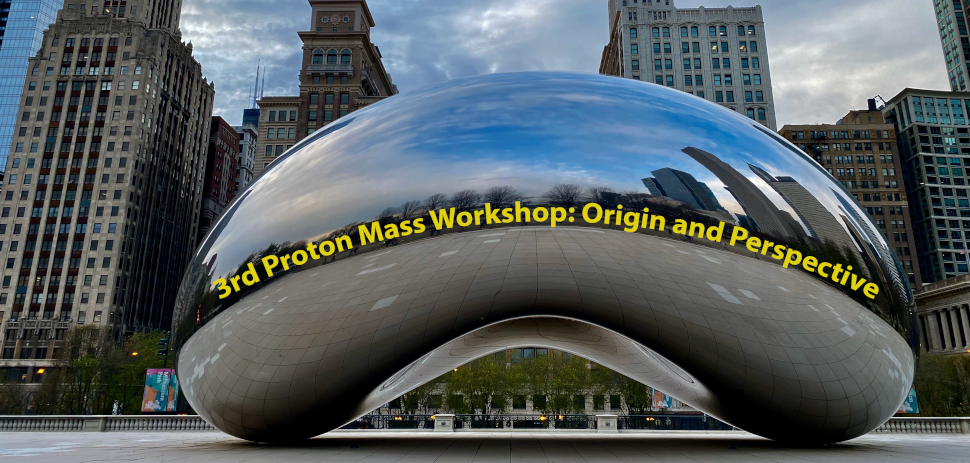#3rd Proton Mass Workshop; Origin and Perspective

Jan 14 – 16, 2021
Argonne National Laboratory
America/New_York timezone

### Paula Dahlberg (Workshop Coordinator)

The next major facility construction in the US is the Electron-Ion Collider planned at Brookhaven National Laboratory. A science assessment by the National Academy of Sciences (https://www.nap.edu/catalog/25171/an-assessment-of-us-based-electron-ion-collider-science) found three central questions that the facility should address, and among these is "How does the mass of the nucleon arise?"

This question goes to the essence of QCD and embodies many of its mysteries and emergent phenomena, such as color confinement. Its understanding is fundamental to the future of nuclear physics, and we think that addressing this question within QCD will expose deep connections and significantly impact the broader fields of cosmology, particle physics, condensed matter, and biology.

In the spirit of the questions posed by Hilbert in the 1900 Mathematics Congress and the Millennium Prize Problems in Mathematical Physics, we would like to identify key theoretical questions to further inform and guide the science program of the Electron-Ion Collider. Our hope is that by posing the right questions this workshop can have a lasting impact on our understanding of the visible universe.

More broadly, this workshop will address and hone key questions related to the proton mass. It is organized around four interelated areas which include the broader fields of physics, the fundamentals of QCD, lattice calculations, hadron models, and last but not least to  experiments and specific observables.

A sample of questions includes:

1. The nucleon mass is the most crucial gravitational charge in the universe below the scale of galaxies. How does the strong interaction scale or mass affect the visible universe?
2. Can the nucleon's transverse momentum parton distributions reveal the temperature at which the nucleon is formed?
3. What determines the QCD scale?
4. What does QCD dynamics predict about the proton mass if the scale is just a parameter?
5. How would the physical world (condensed matter, chemistry, and biology) change if Λ_QCD is increased or decreased by a factor of 2?
6. What is the interplay between the Higgs and QCD mass generation mechanisms?
2. Fundamentals of QCD
1. What is the role of the trace anomaly in QCD? Does it reflect both color confinement and dimensional transmutation?
2. What is the role of chiral symmetry breaking (CSB) in determining the nucleon mass? What is the interplay between the CSB and color confinement?
3. Why are the nucleon resonances (with change of spin and isospin) separated by a large mass gap?
4. Can a relativistic Virial theorem tell us something deeper about the origin of mass?
3. Lattice QCD calculations questions
1. Can one calculate the anomaly contribution on the lattice?
2. Can the lattice calculate the mass distribution in the nucleon?
3. Why is the color magnetic field depleted inside the proton?
4. How does the kinetic energy of quarks balance the color confinement energy of the gluon?
5. Should a glue ball mass be lighter or heavier than the proton mass or 2 pions mass? If so why?